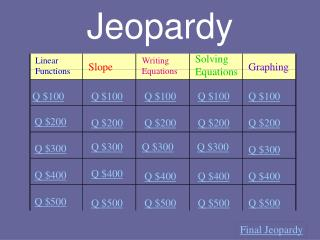DownloadDownload PresentationJeopardy

# Jeopardy

Download Presentation## Jeopardy

- - - - - - - - - - - - - - - - - - - - - - - - - - - E N D - - - - - - - - - - - - - - - - - - - - - - - - - - -
##### Presentation Transcript

1. Jeopardy Solving Equations Linear Functions Writing Equations Slope Graphing Q \$100 Q \$100 Q \$100 Q \$100 Q \$100 Q \$200 Q \$200 Q \$200 Q \$200 Q \$200 Q \$300 Q \$300 Q \$300 Q \$300 Q \$300 Q \$400 Q \$400 Q \$400 Q \$400 Q \$400 Q \$500 Q \$500 Q \$500 Q \$500 Q \$500 Final Jeopardy

2. \$100 Question from Linear Functions Is the following relation a function? Yes or No. (1,3), (1,4), (2,5), (7,6)

3. \$200 Question from Linear Functions State the Domain and Range of The following relation: (1,2), (3,4), (5,6), (7,8)

4. \$200 Answer from Linear Functions D= (1,3,5,7) R= (2,4,6,8)

5. \$300 Question from Linear Functions Given: f(x)= 3x + 3 find f(2)

6. \$400 Question from Linear Functions Given g(x)= 12 – 2x find g(-5)

7. \$500 Question from Linear Functions Given g(x)= 3x +1 and f(x)= 9-2x find: F(2) AND G(3)

8. \$500 Answer from Linear Functions F(2)= 5 G(3) = 10

9. \$100 Question from Slope What is the Slope-Intercept Form of an equation?

10. \$100 Answer from Slope y = mx + b

11. \$200 Question from Slope Find the slope of (1,2) and (3,4)

12. \$300 Question from Slope Write 2y = -2x + 4 in standard form

13. \$300 Answer from Slope 2x + 2y = 4

14. \$400 Question from Slope What is the y – intercept of -2x + 6y = 12

15. \$500 Question from Slope Draw a picture of a line with slope of 0.

16. \$500 Answer from Slope A horizontal line.< ------------------------------- >

17. \$100 Question from Writing Equations Write an equation in Slope-Intercept Form that has a slope of 2 and a y-intercept of 4.

18. \$100 Answer from Writing Equations y = 2x + 4

19. \$200 Question from Writing Equations Write an equation in Standard Form: A = 2 B = -4 C = 9

20. \$200 Answer from Writing Equations 2x – 4y = 9

21. \$300 Question from Writing Equations Write an equation in Slope Intercept form that has a slope of 3 and passes through (1,2).

22. \$300 Answer from Writing Equations y = 3x - 1

23. \$400 Question from Writing Equations Write an equation in Point-Slope Form that has a slope of 4 and passes through (3,2).

24. \$400 Answer from Writing Equations ( y – 3) = 4 ( y – 2)

25. \$500 Question from Writing Equations Write an equation in Slope Intercept Form that passes through (2,3) and (4,5)

26. \$500 Answer from Writing Equations y = x + 1

27. \$100 Question from Solving Equations Solve the following equation: 2x + 5 = 21

28. \$200 Question from Solving Equations Solve the following equation: 4x + 2x + 7 = 12 + 3

29. \$300 Question from Solving Equations Solve the following equation:

30. \$400 Question from Solving Equations Solve: 5(3x + 2) + 10 = -7

31. \$500 Question from Solving Equations Solve the following equation: 4(x + 3) = 3x + 12

32. \$100 Question from Graphing Draw a picture of a line with a positive slope.

33. \$100 Answer from Graphing Slope must be going up (left to right)

34. \$200 Question from Graphing What is the y – intercept of the graph of y = 2x - 1

35. \$300 Question from Graphing Write the slope and the y-intercept of the Following equation of a graph: Y = -8x + 1

36. \$300 Answer from Graphing M = -8 B = 1

37. \$400 Question from Graphing Draw a picture of an undefined slope.

38. \$400 Answer from Graphing VerticalLine

39. \$500 Question from Graphing Find the slope of the line that passes through the following points: (-4, -2), (7, -8), and draw a picture of the slope whether it be positive slope, negative slope, 0 slope, or undefined slope.